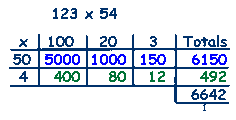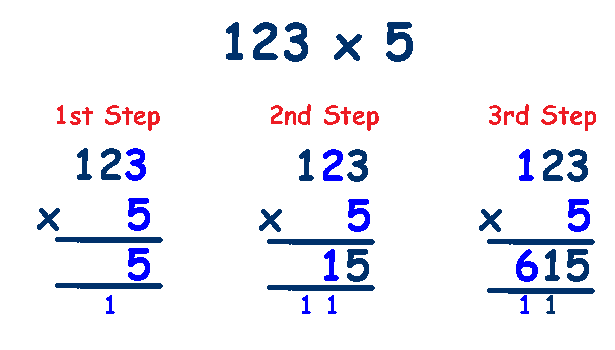# Written methods of Multiplication

This is where a secure knowledge of the times tables to 10 x 10 is essential - if your child has not mastered the times tables yet, you may find this "Multiplication Chart" useful so that they can concentrate on the concept. Don't give up on learning the times tables though - they won't have a chart in the 11 Plus - don't let them get used to using it - throw it away as soon as possible.

Place Value is also very important with multiplication - remind them of the value of the individual digits and review how to multiply and divide easily by 10.

## The Grid Method of Multiplication

First split the number into hundreds, tens and units. (123 is 100, 20 and 3)

Next multiply each of the numbers by 5, which gives 100x5=500, 20x5=100 and 3x5=15.For larger numbers the procedure is the same but with more rows and columns.

First split both numbers into hundreds, tens and units. (123 is 100, 20 and 3 - 54 is 50 and 4).

Next multiply each number by 50 and then 4. Which gives 100x50=5000, 20x50=1000, 3x50=150, 100x4=400, 20x4=80 and 3x4=12.

Then add the rows - 5000+1000+150=6150,

400+80+12=492

Finally add the totals column, 6150+492=6642## The Standard Method of Multiplication

This is the preferred method as it is quicker and takes up less space, every step can be explained by referring to the grid method above.

### We will begin with multiplying HTU by U. (123 x 5)

Write the calculation out correctly, ensuring that the units, tens, hundreds columns are lined up.

First, multiply the units, 3(units) x 5 = 15, 1 ten and 5 units - put the 5 in the units column and the 1 in the tens column below the line.

Second, multiply the tens, 2(tens) x 5 = 10, add the 1 (from step 1) to make 11 (tens), put 1 in the tens column and 1 in the hundreds column below the line.

Lastly, multiply the hundreds, 1(hundreds) x 5 = 5, add this to the 1 already in the 100's column from step 2 to make 6 and write this in the hundreds column.### Next we will move on to HTU by TU. (123 x 45)

Write the calculation out correctly, ensuring that the units, tens, hundreds columns are lined up.

The first step is to multiply 123 by 5 (the units) exactly as in the previous example.

Now we have to multiply 123 by 40 (the tens)
It is important to remember that any number multiplied by any multiple of 10 (10,20,30,40 etc) always results in the unit digit being a zero. So we put a 0 in the unit column and then we only have to multiply 123 by 4.

Now we can proceed to multiply 123 by the tens, 123 x 4, putting the answers into the correct columns of the next row. 3x4, then 2x4 and finally 1x4 to give 123 x 40 = 4920.

Lastly, we add the two multiplications together,
615 + 4920 to give 5535.### Full screen version

Diamond Mining - A great worksheet to practice written multiplication.

HTU x U written multiplication worksheet from Mathsblog.

HTU x TU written multiplication worksheet from Mathsblog.